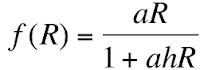# Equation of the Month

A blog run by the

Theoretical Population Ecology and Evolution Group,

Biology Dept.,

Lund University

The purpose of this blog is to emphasize the role of theory for our understanding of natural, biological systems. We do so by highlighting specific pieces of theory, usually expressed as mathematical 'equations', and describing their origin, interpretation and relevance.

## Friday, August 31, 2012

### The Disc EquationWhat it means
The disc equation models the rate of intake (f) of prey items (with population density R) by a single predator while its only activity is foraging (searching for and handling prey). The two parameters are a, the attack (or search) rate (a constant) and h, the handling time per prey (also a constant). This function increases monotonically towards an asymptote set by 1/h. The shorter the handling time per prey, the higher the maximum intake rate. The disc equation is an example of a functional response model.

Compare this equation with the Monod equation (bacterial growth) and the Michaelis-Menten equation (for enzymatic reaction rates).

Where does it come from?
In 1959, C. S. Holling published two seminal papers on the ”functional response” of predators, i.e., how the rate of predation should vary with prey density. In the first paper, Holling (1959a) figures out four types of predation, the second one (”Type II”) was elaborated on and derived more formally in the second paper (Holling, 1959b). It was subsequently named the ”disc equation” because the experiment Holling set up was done using artificial food items on a sandpaper disc and a blindfolded person (his secretary, no less) ”predating” on them. Holling noted that for a given rate of attack (a), more and more of the total time was spent handling prey as prey density increased, and that the intake rate therefore should be discounted by the handling time per prey item. Holling’s real interest was to understand how and when predators can regulate the density of prey species, particularly forest pest insects.

Importance
The disc equation soon became the standard model in practically all studies of foraging behavior and predator-prey interactions. The model was supported by numerous experimental results on a wide variety of predators feeding on a single prey type. With more than one type to feed on, the disc equation has been extended to the multiple prey functional response (Murdoch and Oaten 1975). That model assumes that the predator has no preference for any given prey type. If that is the case, the disc equation is less useful and models with switching rules and prey type preferences are better suited for the problem. The disc equation was used in the Rosenzweig-MacArthur model in 1963 and it quickly became the standard alternative to the linear Lotka-Volterra model of predator-prey dynamics.

Since the disc equation models a decelerating intake rate with increasing prey density, it leads to prey safety in numbers – the higher the prey density, the lower the per capita risk of being eating. This tends to destabilize predator-prey interactions.

The disc equation is still a backbone of foraging theory and in theories of predator-prey interactions and food web dynamics.

Per Lundberg

Literature
Holling, C. S. 1959a. The Components of Predation as Revealed by a Study of Small-Mammal Predation of the European Pine Sawfly. The Canadian Entomologist 91: 293-320.

Holling, C. S. 1959b. Some characteristics of simple types of predation and parasitism. The Canadian Entomologist 91: 385-398.

Murdoch, W. W and Oaten, A. 1975. Predation and population stability. Adv. Ecol. Res. 9: 1-131.

Rosenzweig, M. L. and MacArthur, R. H. 1963. Graphical representation and stability conditions of predator-prey interactions. Am. Nat. 97: 209-223.